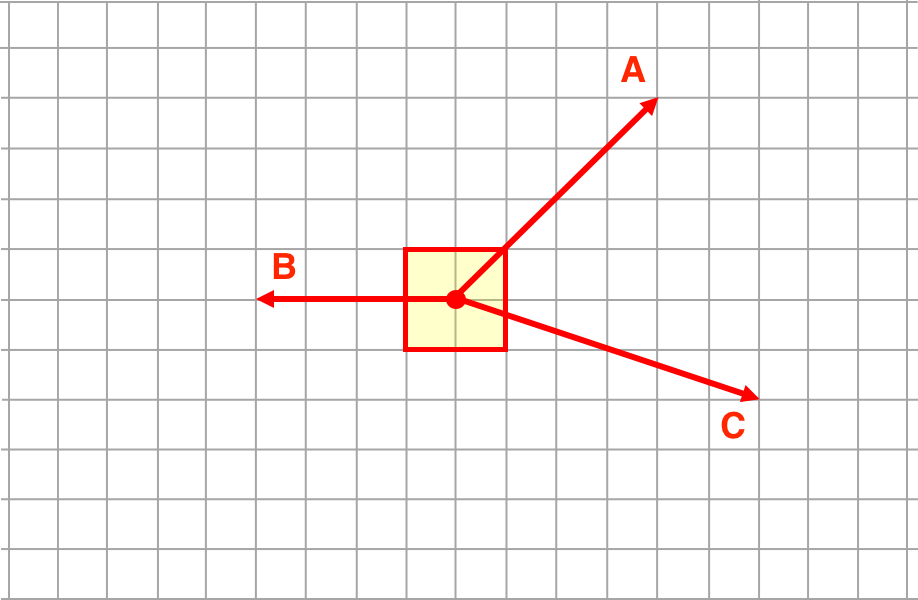#### The Basic Idea

When all the individual forces acting upon an object are balanced, then the object is at equilibrium. When a force is at an angle to the traditional x-y axes, it is customary to consider the components of the force in the analysis. The components of a force vector are the projections of that vector onto the x- and the y-axis. By thinking in terms of components, it is considerably easier to determine what forces would be required to bring an object to equilibrium.

There are a total of 60 Questions in this activity. Eave level includes three questions that are picked at random. As such it is impossible to predict which question prompted you to click the Help Me! button and arrive at this Help page. An example question is shown below.

Version 1:
Consider the three angled forces below. Add one E-W force and one N-S force so that the object is at equilibrium.In this problem, you want to balance the given force(s) by adding two forces to the object. At least one of the forces is an angled forcs. An angled force has an  x- and a y-component. Since the forces are drawn on a background grid of squares that represent 10 N along each edge, it is easy to determine the x- and the y-components.  For instance, an angled force vector that stretches horizontally for 7 squares has an x-component of 70 N.  If the same angled vector stretches vertically across 4 squares, then the y-component is 40 N. Begin this problem by determining the x- and the y-component of the given force(s).

"Vector Summing" the Forces
The next step is to add the components of the given forces (assuming there is more than one given force). Components are vectors too so when you add components you must consider their direction. Add the x-component of the given forces to get the total amount of x-Force provided by the given force vectors. Repeat the process for the y-components; add the y-components of the given force vectors to determine the total amount of y-Force. (And by the way, it doesn't hurt to have a scratch pad by your side to write some numbers down.)

Balance the Forces
If the given forces have a combined (sum) x-component of 70 N to the west, then you need to add an equal strength force in the opposite direction. That is the only way that you can balance the given forces. So add a force of 70 N to the east. By the same reasoning, you will have to add a vertical force to balance the combined (sum) y-component of the given forces. So if the combined  y-component is 40 N to the north, then add a 40 N force directed southward.